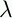September 22, 1999 Perl and the Lambda Calculus Slide #17

# Currying

For notational convenience with curried functions, we allowxy.E

as an abbreviation forx.y.E

Example:

(xy.(x (x y)) P Q)

(y.(P (P y)) Q)

```
```

(P (P Q))

 NextCopyright © 1999 M-J. Dominus